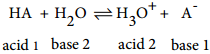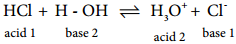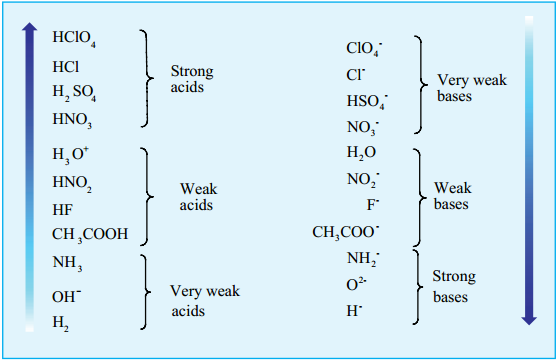Find free online Chemistry Topics covering a broad range of concepts from research institutes around the world.

## Strength of Acids and Bases

The strength of acids and bases can be determined by the concentration of H3O+ (or) OH produced per mole of the substance dissolved in H2O. Generally we classify the acids/bases either as strong or weak. A strong acid is the one that is almost completely dissociated in water while a weak acid is only partially dissociated in water.

Let us quantitatively define the strength of an acid (HA) by considering the following general equilibrium.The equilibrium constant for the above ionisation is given by the following expression………… (8.1)

We can omit the concentration of H2O in the above expression since it is present in large excess and essentially unchanged.……………. (8.2)

Here, Ka is called the ionisation constant or dissociation constant of the acid. It measures the strength of an acid. Acids such as HCl, HNO3 etc… are almost completely ionised and hence they have high Ka value (Ka for HCl at 25°C is 2 × 106).

Acids such as formic acid (Ka = 1.8 × 10-4 at 25°C), acetic acid (1.8 × 10-5 at 25°C) etc.. are partially ionised in solution and in such cases, there is an equilibrium between the unionised acid molecules and their dissociated ions. Generally, acids with Ka value greater than ten are considered as strong acids and less than one considered as weak acids.

Let us consider the dissociation of HCl in aqueous solution,As discussed earlier, due to the complete dissociation, the equilibrium lies almost 100% to the right. i.e., the Cl ion has only a negligible tendency to accept a proton form H3O+. It means that the conjugate base of a strong acid is a weak base and vice versa. The following table illustrates the relative strength of conjugate acid – base pairs.